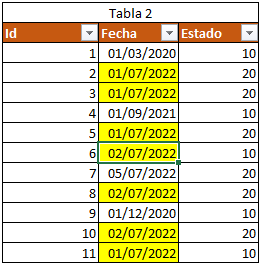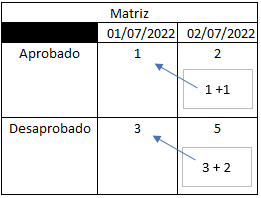cancel
Showing results for
Did you mean:## Cumulative count in matrix filtered by date

Good afternoon, I would appreciate it very much if you can help me

The case is as follows:

I have two tables with data

The first table is:from where we will take the categories for the matrix.

The second table is:From this we will take those that are from July 1 and 2, 2022.

What I am looking for is to be able to make the following matrix:What I need is for July 2, 2022 to add the value of the previous day plus the value of July 2.

Please note that from table 2 there is data from other dates which I do not want to bring to the matrix and that I would prefer to do it within the DAX formula and not with external filters.

One more doubt: if you wanted to bring the accumulated of the previous month, that is, the figure of 30/06/2022, it can be done and how it would be done???.

I thank you for the yuda.

2 REPLIES 2Super User

You can do something like

``````cumulative sum =
CALCULATE (
SUM ( table[value] ),
FILTER (
ALL ( table[date] ),
table[date] <= MAX ( table[date] )
) //to create a sum of values where  date is less than or equal to the date in the current filter context
)``````

Proud to be a Super User!

"Tell me and I’ll forget; show me and I may remember; involve me and I’ll understand."
Connect to me on LinkedIn || Need consulting? Hire me for a Power BI gig on UpWork.